## Steady-State Brusselator with Diffusion

The system is the same as the one in WOBexa1. Scaling the independent variable according to t:=x/L leads to the system of four ODEs of the first order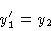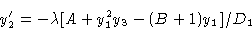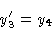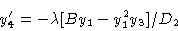with boundary conditions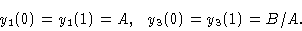The ordinary differential equations describe the spatial dependence of the two chemicals X and Y along a reactor with length L,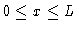. This Brusselator model equation has a great number of solutions ; some are represented in the branching diagram of Figure 1, which depicts y2(0)=X'(0) versus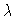. One nontrivial bifurcation point is found for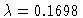, y2(0)=6.275.

Figure 1
Bifurcation diagram X'(0) versusThis Example
Figure 1